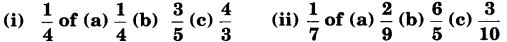# Class 7 Maths NCERT Solutions for Chapter 2 Fractions and Decimals EX – 2.3

## Fractions and Decimals

Question 1.
Find :Solution:

Question 2.
Multiply and reduce to lowest form (if possible) :Solution:

Question 3.
Multiply the following fractions :Solution:

Question 4.
Which is greater?Solution:

Question 5.
Saili plants 4 saplings in a row, in her garden. The distance between two adjacent saplings is$\frac { 3 }{ 4 }$ m. Find the distance between the first and the last sapling.

Solution:
Let four saplings be planted in a row at the points A, B, C and D such that AB = BC = CD =$\frac { 3 }{ 4 }$ mQuestion 6.
Lipika reads a book for 1$\frac { 3 }{ 4 }$ hours everyday. She reads the entire book in 6 days. How many hours in all were required by her to read the book?

Solution:

Question 7.
A car runs 16 km using 1 litre of petrol. How much distance will it cover using 2$\frac { 3 }{ 4 }$ litres of petrol?

Solution:

Question 8.
(a) (i) Provide the number in the box ( ), such that$\frac { 2 }{ 3 }$ × ( ) =$\frac { 10 }{ 30 }$
(ii) The simplest form of the number obtained in ( ) is __________
(b) (i) Provide the number in the box ( ) such that$\frac { 3 }{ 5 }$ × ( ) =$\frac { 24 }{ 75 }$
(ii) The simplest form of the number obtained in ( ) is __________

Solution: